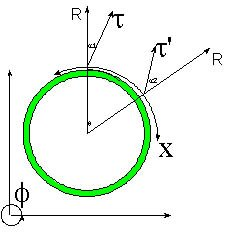# Hypergeometrical Universe

## Universe as a Higher Dimension Shock WaveUniverse as a Higher Dimension Shock Wave

Many people might have a knee-jerk reaction to the premises of the HyperGeometrical Universe Theory of Everything (HU for simplicity)...

There are undoubtedly new ideas which are tough to digest. We are all used to think the same thoughts and new concepts are supposed to receive higher scrutiny. I will try to clarify some of the most likely contentious points of the theory:

1. The first that comes to mind is the iconoclastic idea that we are traveling at the speed of light from the focal point of a four-dimensional space (or five dimensional spacetime) Big Bang.
The Big Bang has been proposed to be the result of a four-dimensional Big Bang in the past. Li and Wesson proposed this in his paper
"The Big Bang as a higher-dimensional shock wave". In this paper the three dimensional Universe is presented as a hypersurface immersed on the fifth dimension. Solution for Einstein field equations without sources are provided including Wave-Like solutions of RAB=0. The topology is a one of a matter filed thus curved 3-D space in a flat 4-dimensional space. This means that the curvature is mass derived and the Universe resembles more an sphere inside a swimming pool. The Big Bang shock wave can be thought as 3-D shock wave crossing a page-like (flat) Universe.

The crossing of the 4D shock-wave on our 3D cross-section creates what I consider an unpalatable anisotropy. Just a matter of taste.
In my theory, the shock wave creates not a flat Universe curved by its mass, but a 3-D shell-like expanding at the speed of light.
The 3D Universe is the Shock Wave.
This is a tremendous departure from a 4D Shock wave disturbing a 3D cross-section...:) and of course, it is where Li and Wesson missed the boat. Not unlike everyone else, their space curvature is actually spacetime curvature and it is considered to be due to Mass - copycatting Einstein..>:)
In my theory the curvature is space curvature and it doesn't depend upon mass distribution...:) This curvature was never considered because nobody came up with a non-compact topology like the one I proposed. Simple as that...:)
It is difficult to be precise when speaking about curvature along a dimension we are not used to think about. I will only mention that there might be differences when I speak of curvature and when Relativity speaks of curvature. The other point is that the 3-D shell is defined based on the figure above, that is, X, Y and Z are perfect circles. As we travel around the Universe, we are always in the hyperspherical shock wave and if it were possible to propel oneself at speeds higher than the speed of light we would be able to go around the Universe. In fact, we would be able to get out of the Universe into the Void.
One might ask why the speed of light? The answer is simple and based on the following points:
1. Black Holes are considered to be very curved spacetime created by accretion of mass.
2. If we fall in a Black Hole we will be one with it, thus we will be a deformation of spacetime.
3. If item 2 is correct, we should be a deformation of spacetime since the beginning.
4. Light is due to alternate motion of matter (electrons), thus it should be a propagating deformation of spacetime.
5. Light propagates at the speed of light.
6. We are a non-constrained deformation of spacetime and thus we also should propagate at the speed of light.
7. If we are not traveling at the speed of light, that should mean that we are traveling perpendicularly to the directions we are able to see, thus we should be traveling perpendicular to our 3-D Universe.
8. The fact that we don't see anything from outside this Universe means that there shouldn't be any dilator (generator of spacetime oscillations) outside our 3-D shell.
9. The topology should be hyperspherical or whatever one would call this 4-dimensional object I proposed in my papers...:)
10. The space is naturally curved and has a radius of curvature equal to the dimensional age of the Universe (c * the age of the Universe).
It is important to notice that prior 4-Dimensional proposals of Big Bang as a Higher Dimensional Shock Wave missed the possibility that the Universe itself would be the shock wave.
Currently unrated

or

RSS / Atom